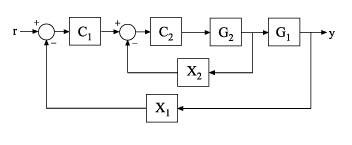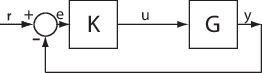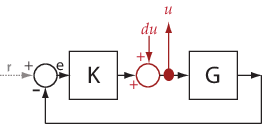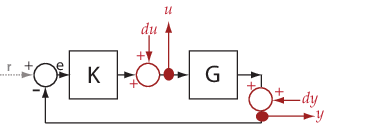Documentation

getSensitivity

Sensitivity function from generalized model of control system

Description

example

S = getSensitivity(T,location) returns the sensitivity function at the specified location for a generalized model of a control system.

example

S = getSensitivity(T,location,opening) specifies additional loop openings for the sensitivity function calculation. Use an opening, for example, to calculate the sensitivity function of an inner loop, with the outer loop open.

If opening and location list the same point, the software opens the loop after measuring the signal at the point.

Examples

collapse all

Compute the sensitivity at the plant input, marked by the analysis point X.Create a model of the system by specifying and connecting a numeric LTI plant model G, a tunable controller C, and the AnalysisPoint block X. Use the AnalysisPoint block to mark the location where you assess the sensitivity (plant input in this example).

G = tf(,[1 5]);
C = tunablePID('C','p');
C.Kp.Value = 3;
X = AnalysisPoint('X');
T = feedback(G*X*C,1);

T is a genss model that represents the closed-loop response of the control system from $r$ to $y$. The model contains the AnalysisPoint block, X, that identifies the analysis point.

Calculate the sensitivity, $S$, at X.

S = getSensitivity(T,'X');
tf(S)
ans =

From input "X" to output "X":
s + 5
-----
s + 8

Continuous-time transfer function.

Calculate the inner-loop sensitivity at the output of G2, with the outer loop open.Create a model of the system by specifying and connecting the numeric plant models, tunable controllers, and AnalysisPoint blocks. G1 and G2 are plant models, C1 and C2 are tunable controllers, and X1 and X2 are AnalysisPoint blocks that mark potential loop-opening locations.

G1 = tf(10,[1 10]);
G2 = tf([1 2],[1 0.2 10]);
C1 = tunablePID('C','pi');
C2 = tunableGain('G',1);
X1 = AnalysisPoint('X1');
X2 = AnalysisPoint('X2');
T = feedback(G1*feedback(G2*C2,X2)*C1,X1);

Calculate the sensitivity, $S$, at X2, with the outer loop open at X1.

S = getSensitivity(T,'X2','X1');
tf(S)
ans =

From input "X2" to output "X2":
s^2 + 0.2 s + 10
----------------
s^2 + 1.2 s + 12

Continuous-time transfer function.

Input Arguments

collapse all

Model of a control system, specified as a generalized state-space model (genss).

Locations at which you can perform sensitivity analysis or open loops are marked by AnalysisPoint blocks in T. Use getPoints(T) to get the list of such locations.

Location at which you calculate the sensitivity function, specified as a character vector or cell array of character vectors. To extract the sensitivity function at multiple locations, use a cell array of character vectors.

Each specified location must match an analysis point in T. Analysis points are marked using AnalysisPoint blocks. To get the list of available analysis points in T, use getPoints(T).

Example: 'u' or {'u','y'}

Additional loop opening used to calculate the sensitivity function, specified as a character vector or cell array of character vectors. To open the loop at multiple locations, use a cell array of character vectors.

Each specified opening must match an analysis point in T. Analysis points are marked using AnalysisPoint blocks. To get the list of available analysis points in T, use getPoints(T).

Use an opening, for example, to calculate the sensitivity function of an inner loop, with the outer loop open.

If opening and location list the same point, the software opens the loop after measuring the signal at the point.

Example: 'y_outer' or {'y_outer','y_outer2'}

Output Arguments

collapse all

Sensitivity function of the control system, T, measured at location, returned as a generalized state-space model (genss).

• If location specifies a single analysis point, then S is a SISO genss model.

• If location is a vector signal, or specifies multiple analysis points, then S is a MIMO genss model.

collapse all

Sensitivity Function

The sensitivity function, also referred to simply as sensitivity, measures how sensitive a signal is to an added disturbance. Feedback reduces the sensitivity in the frequency band where the open-loop gain is greater than 1.

Consider the following model:The sensitivity, Su, at u is defined as the transfer function from du to u:$\begin{array}{l}u=du-KGu\\ \to \left(I+KG\right)u=du\\ \to u=\underset{{S}_{u}}{\underbrace{{\left(I+KG\right)}^{-1}}}du.\end{array}$

Here, I is an identity matrix of the same size as KG.

Sensitivity at multiple locations, for example, u and y, is defined as the MIMO transfer function from the disturbances to sensitivity measurements:$S=\left[\begin{array}{cc}{S}_{du\to u}& {S}_{dy\to u}\\ {S}_{du\to y}& {S}_{dy\to y}\end{array}\right].$